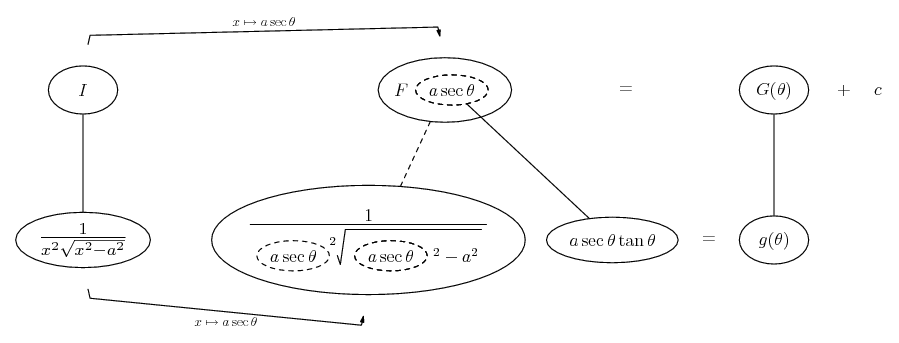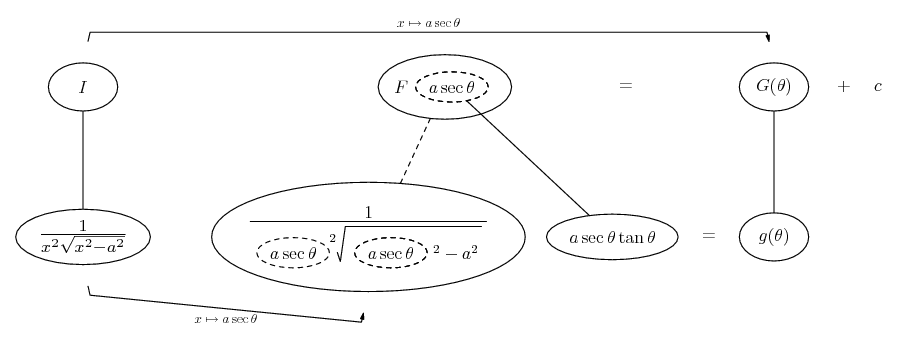# Thread: use trig substitution

1. ## use trig substitution

show that integral 1/x^2squareroot(x^2-a^2) =
((squareroot(x^2-a^2))/a^2x)+C using a trigonometric substitution

2.Originally Posted by mikegar813show that integral 1/x^2squareroot(x^2-a^2) =
((squareroot(x^2-a^2))/a^2x)+C using a trigonometric substitution
I remember that $\displaystyle sin^2(\theta)+ cos^2(\theta)= 1$ so that, dividing by $\displaystyle cos^2(\theta)$, $\displaystyle tan^2(\theta)- 1= sec^2(\theta)$ and then $\displaystyle a^2tan^2(\theta)- a^2= a^2sec^(\theta)$.

That suggests using the substitution $\displaystyle x= a tan(\theta)$ to get a "perfect square" inside the square root.

3.Originally Posted by HallsofIvyI remember that $\displaystyle sin^2(\theta)+ cos^2(\theta)= 1$ so that, dividing by $\displaystyle cos^2(\theta)$, $\displaystyle tan^2(\theta)- 1= sec^2(\theta)$ and then $\displaystyle a^2tan^2(\theta)- a^2= a^2sec^(\theta)$.

That suggests using the substitution $\displaystyle x= a tan(\theta)$ to get a "perfect square" inside the square root.
Typo... HoI surely means

dividing by $\displaystyle cos^2(\theta)$, $\displaystyle tan^2(\theta) + 1= sec^2(\theta)$ and then $\displaystyle a^2 \sec^2(\theta) - a^2 = a^2 \tan^2(\theta)$.

That suggests using the substitution $\displaystyle x= a \sec(\theta)$ to get a "perfect square" inside the square root

Edit:

Just in case a picture helps...... where... is the chain rule. Straight continuous lines differentiate downwards (integrate up) with respect to x, and the straight dashed line similarly but with respect to the dashed balloon expression (the inner function of the composite which is subject to the chain rule).

Carry on, anti-clockwise... g(theta) should be a nice simplification with a straightforward integral G. Then use a triangle or whatever to map back to x. Actually, as F(a sec theta) = G(theta) you can map from G..._________________________________________

Don't integrate - balloontegrate!

http://www.ballooncalculus.org/examples/gallery.html

Draw balloons with LaTeX: http://www.ballooncalculus.org/asy/doc.html

#### Search Tags

substitution, trig